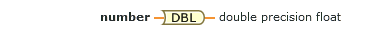# To Double Precision Float (G Dataflow)

Converts a number to a double-precision, floating-point number.## number

An input to this operation.

This input supports waveforms and any data type that contains only numbers, such as scalar numbers, arrays or clusters of numbers, and arrays of clusters of numbers.

Data Type Changes on FPGA

When you add this node to a document targeted to an FPGA, this input has a default data type that uses fewer hardware resources at compile time.## double precision float

Result of the conversion.

This output assumes the same data type structure as number.

Where This Node Can Run:

Desktop OS: Windows

FPGA: DAQExpress does not support FPGA devices